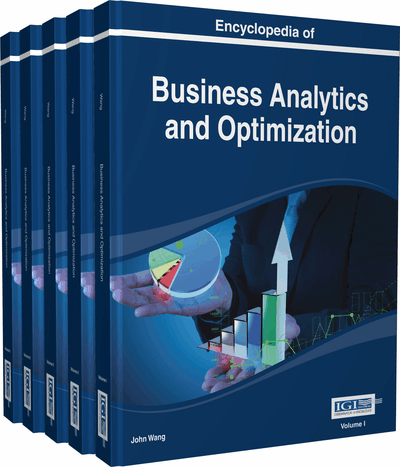# Empirical Likelihood-Based Method for LME Models

Haiyan Su (Montclair State University, USA)
DOI: 10.4018/978-1-4666-5202-6.ch076

Top

## Introduction

Longitudinal data are often encountered in biomedical, epidemiological, social science and business studies. Repeated measurements are made on subjects over time and responses within a subject are usually correlated. The interest of longitudinal study is usually the growth curve over time. Linear mixed-effects models (LME) are widely used for longitudinal data because it allows both the population level fixed effects and individual level random effects to appear linearly in the model linearly in the model (Pinheiro & Bates, 2000). In modeling the fixed effects and random effects coefficients, the normality assumption of the random error terms and the random effect terms are required. Violation of this assumption may yield invalid statistical inference. Since the normality assumption is too restrictive as pointed out by Zhang and Davidian (2001, broader classes of distributions for the mixed models have been proposed by Verbeke and Lesaffre (1997), Zhang and Davidian (2001), Chen et al., (2002) among others. Ma and Genton (2004) relaxed the normality assumption by using semiparametric generalized skew-ellipitical distributions. Zhou and He (2008) replaced the normal distribution with skew-t distributions. Lachos et al., (2009) proposed a Bayesian approach with asymmetric heavy tailed distribution. The generalized estimation equation (GEE) (Liang & Zeger, 1986) approach relaxed this assumption by focusing on population-level effects using the quasi-likelihood method. GEE is a very popular approach researcher use when normality assumption is violated for continuous responses and it is supported by most available software such as SAS and R .

In this paper, we develop an empirical likelihood-based method to get inference for population level parameter of interest in linear mixed-effects models when normality assumption is violated. Empirical likelihood was introduced by Owen (1988, 1990). It amounts to computing the parametric likelihood of a general multinomial distribution which has its atoms at all observed data points. Empirical likelihood provides a good alternative among the nonparametric methods that can be used to make statistical inference when the normality assumption is violated. The advantage of empirical likelihood compared to the bootstrap method and the jackknife method arises because it is a nonparametric method of inference based on a data-driven likelihood ratio function. A thorough summary of the advantages of empirical likelihood over its competitors is given in Hall and La Scala (1990). In this study, we suggest doing a projection to erase nuisance parameters for dimension reduction and then using the profile empirical likelihood principle. We then apply the Bartlett correction method to obtain the adjusted confidence interval. The paper is organized as follows: We give the main results including the model formulation, the Bartlett correction procedures and proofs of the theoretical results in the “Main Focus” section. Then follows results from simulation studies by comparing the proposed method with normal approximation method and the GEE method and a real application study. We then give the conclusion of the paper and the future trends.

## Key Terms in this Chapter

Coverage Probability: In statistics, the coverage probability of a confidence interval is the proportion of the time that the interval contains the true value of interest.

Fixed Effects: In panel data analysis, the term fixed effects estimator is used to refer to an estimator for the coefficients in the regression model. If we assume fixed effects, we impose time independent effects for each entity that are possibly correlated with the regressors.

Nuisance Parameters: A nuisance parameter is any parameter which is not of immediate interest but which must be accounted for in the analysis of those parameters which are of interest.

Empirical Likelihood: Empirical likelihood allows the statistician to employ likelihood methods, without having to pick a parametric family for the data. It is described in two articles published by Owen (1988 , 1990 ) and a monograph published by Chapman and Hall/CRC Press (ISBN 1584880716) ( Owen, 2001 ).

Bartlett Correction: A Bartlett correction is a scalar transformation applied to the likelihood ratio (LR) statistic that yields a new, improved test statistic which has a chi-squared null distribution to order O(1/n). This represents a clear improvement over the original statistic in the sense that LR is distributed as chi-squared under the null hypothesis only to order O(1).

Linear Mixed-Effects Models: A linear mixed model is a statistical model that responses from a subject are thought to be the sum of fixed and random effects, which is mixed effects. These models are useful in a wide variety of disciplines in the physical, biological and social sciences. They are particularly useful in settings where repeated measurements are made on the same statistical units, or where measurements are made on clusters of related statistical units.

Confidence Interval: In statistics, a confidence interval (CI) is a kind of interval estimate of a population parameter and is used to indicate the reliability of an estimate.

## Complete Chapter List

Search this Book:
Reset